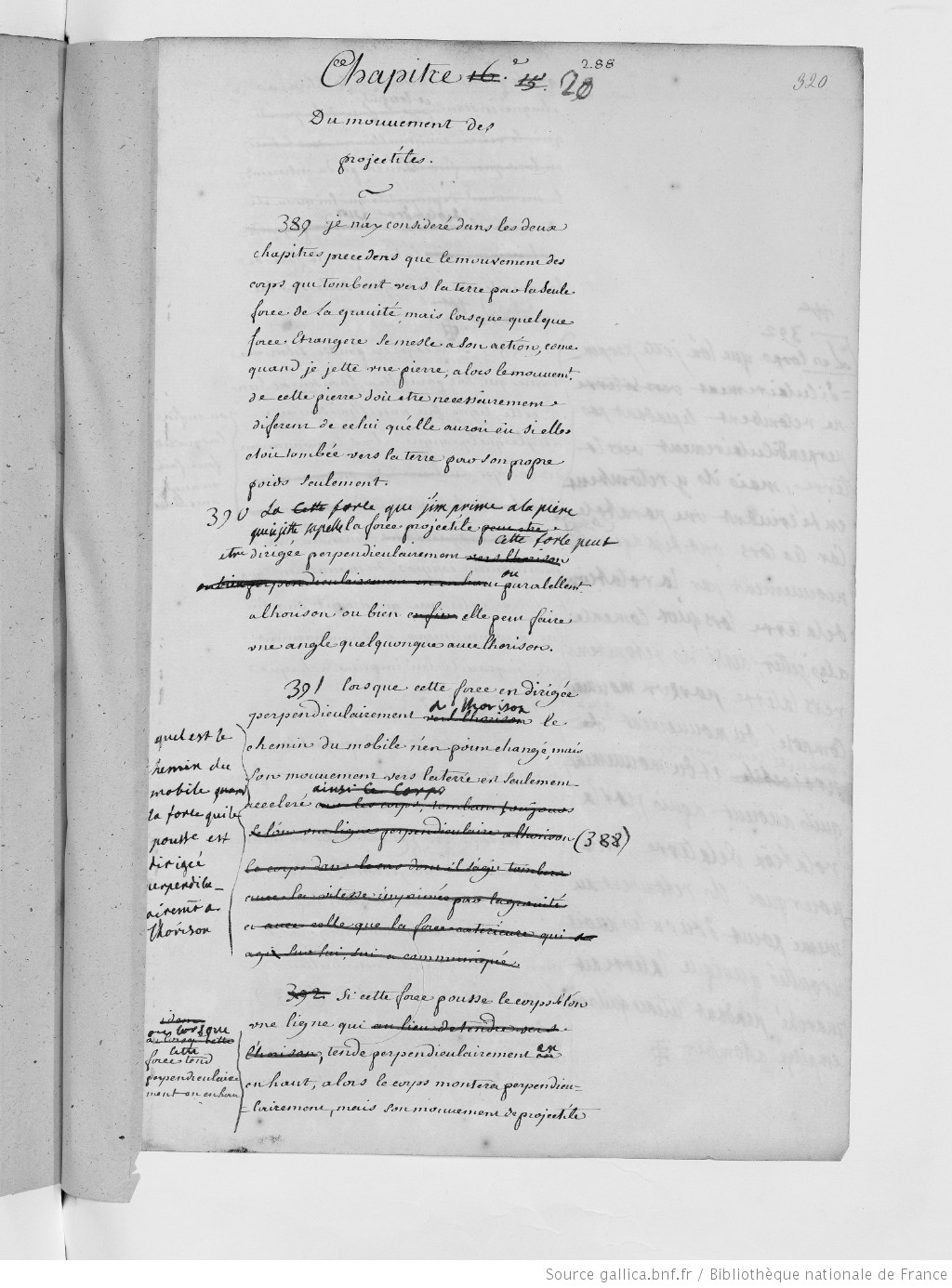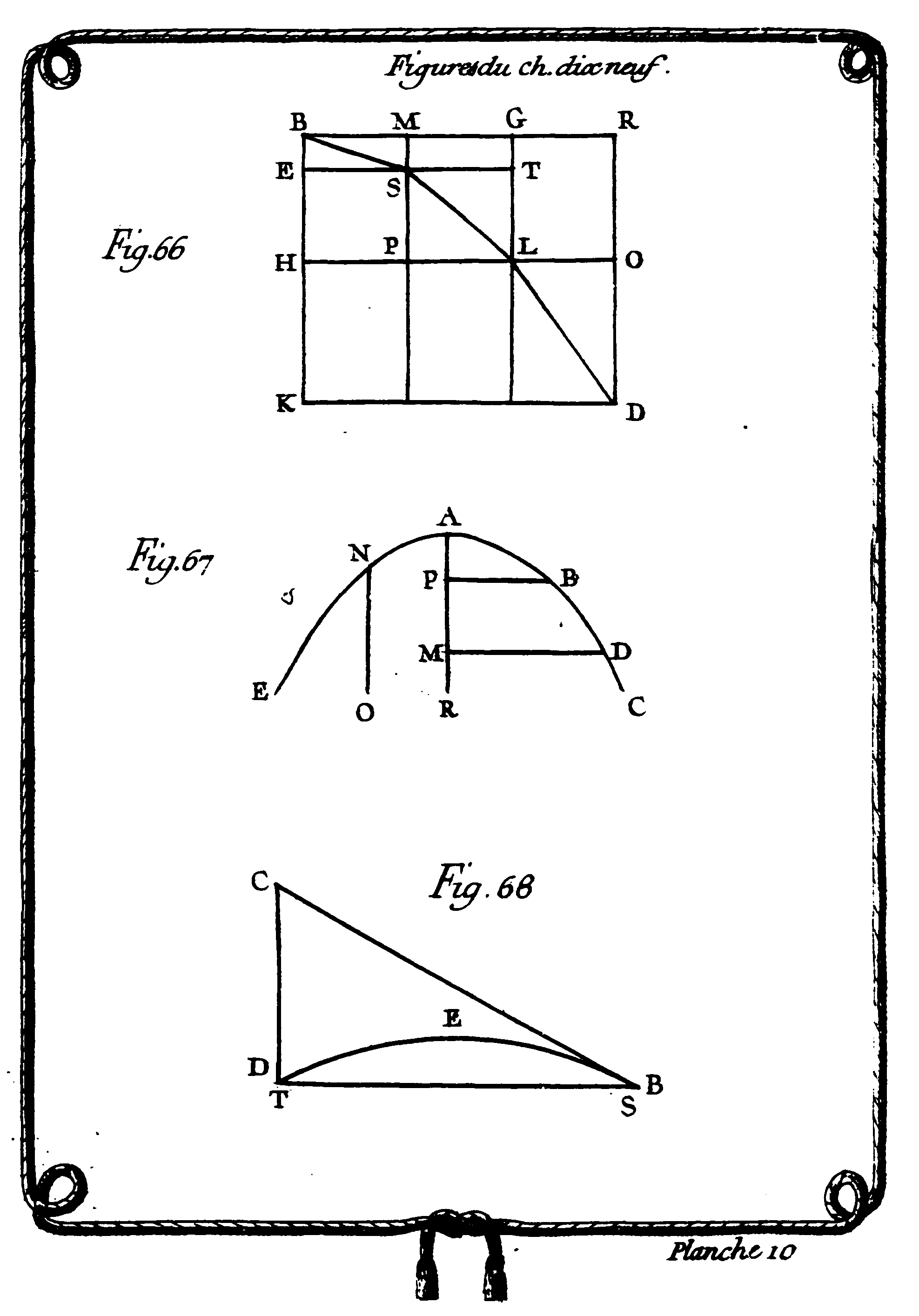Chapter 19. Of the Motion of ProjectilesThis chapter deals with a special case of the motion of bodies, namely with projectile motion. Projectile motion is a form of motion experienced by a thrown object (a projectile) along a curved path. The study of such motions is called ballistics. In today’s teaching cources it is taught that the only force of significance that acts on the object is gravity. By contrast, Du Châtelet introduces the projectile force. It is described as a specific kind of an impressed force that can be directed perpendicularly or in parallel to the horizon, or indeed it can make any angle with it (InstPhy, § 502).

What Du Châtelet really describes is, from today’s point of view, the change in momentum, or impulse. (A force acting for a given amount of time will change an object’s momentum.) Du Châtelet then analyses the curve of a projectile in a non-resisting medium for cases of vertical, horizontal and oblique throws. In all three cases, the line that the body describes when it is thrown in a direction vertical or oblique to the horizon is a parabola. In § 507, Fig. 66, Du Châtelet explains, for example, the throwing parabola from B to D when the projectile is thrown in a horizontal direction (the direction of the projectile force is parallel to the horizon). In § 513, Fig. 68, the “amplitude” and the “angle of elevation” are defined as follows:The parabola BED is called the path of the moveable body, and the right line ST which subtends this parabola BD described by this body in its motion, is called the amplitude of this path, and the angle CBT is called the angle of elevation. (Copyright © 2018 KB)

Another example for Du Châtelet’s discussion of projectile trajectories is the following one (InstPhy, § 511; Fig. 67): One of the properties of the parabola is that the parameter of its axis or of one of its diameters is the third proportional to the abscissa of this diameter and its ordinate. If one knows it’s distance in a given time, then one can describe the path of the body.

The geometrical idealization of projectile trajectories allows to neglect physical effects, like air resistance. Strictly speaking, the body follows an elliptical Kepler orbit, not a parabola (InstPhy, § 516):

With regard to the resistance of air to vertical and horizontal motion, that one supposes to be zero when one determines that the curve described by falling projectiles is a parabola, its effect is so sensible in the fall of ordinary bodies that the curve that they describe when falling in the air is no longer a parabola, but a curve closely approaching a hyperbola, which receives alterations according to the mass and the form/shape of the bodies, and according to the nature of the air in which they fall. (Copyright © 2018 KB)

This proposition dates back to Newton’s Principia, Book 2, Proposition 4, where Newton defined the motion of a projectile which suffers resistance proportional to its velocity. To support the assertion that air resistance is neglegible for practical purposes like in the art of artillery, Du Châtelet refers to François Blondel’s treatise on L’Art de jetter les bombes (1683), in which he addressed the problem of air resistance and claimed that it can be ignored.

##### Back to main project | Next chapter